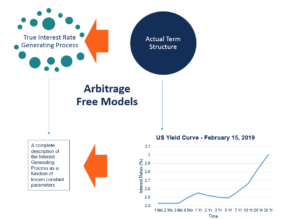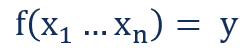# Arbitrage Free Term Structure Models

Models used to generate the true stochastic interest rate generating process by using real market data

## What are Arbitrage Free Term Structure Models?

Arbitrage Free Term Structure Models (also known as No-Arbitrage Models) are used to generate the true stochastic interest rate generating process by using real market data. Unlike equilibrium term structure models, which make certain assumptions about the true interest rate generating process to determine the correct theoretical term structure, arbitrage free term structure models use the actual term structure (known as the realized term structure) to estimate the true interest rate generating process.Arbitrage free term structure models are less theoretical models and are more exercises in data fitting. They are known as arbitrage free because they work under the assumption that the market term structure is correct and that there are no opportunities for arbitrage.

### Arbitrage Free Term Structure Model Parameters

Arbitrage free term structure models usually describe the interest rate process as a function of constant parameters. The accuracy of the data fitting exercise increases with the number of parameters and in general, the following result holds in the population:Where:

• y is the true description of the term structure

While working with sample data, an arbitrage free term structure model can be made to fit the data exactly by using as many constant parameters as data points. In practice, a small number of constant parameters are usually enough to provide a good approximation of the term structure. A subtle and often overlooked property of arbitrage free term structure models is that numerically solving the stochastic process generated by an arbitrage free model will always necessarily yield the term structure as output.

Mathematically, it implies that the data fitting exercise behind arbitrage free models is, in some sense, one-to-one mappings. The result, which some consider purely definitional, drastically reduces the time needed to solve the models using numerical optimization techniques.

### The Ho-Lee Model

The Ho-Lee Model was the first arbitrage free term structure model. It was introduced by Thomas Ho and Sang Bin Lee in their 1986 paper, “Term Structure Movements and Pricing Interest Rate Contingent Claims.” The Ho-Lee Model parameterizes the term structure using only two parameters:

• The standard deviation of the short-term interest rate.
• The term premium of the short-term interest rate.